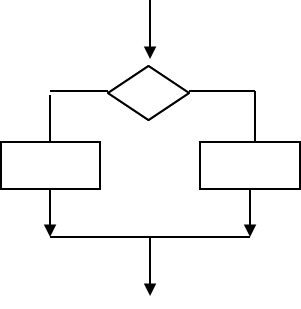# 6 Coding Puzzles Teach You How to Read IF statements

This blog continues our series of C# coding brainteasers, which teach you how to read code without compiling it. This blog will provide 6 puzzles on IF statements. The puzzles will help you learn practical programming skills.This is how an IF statement works.  An IF statement runs code based on a test. This test results in true or false. When it results in true on or more statements run. Optionally alternative statements can run if the test results in false. IF statements are also called conditional statements, since code is executed based on value of the condition (test). IF statements are frequently used in all levels of programming.

Try to solve these 6 IF statements with your C# or other coding knowledge. Just predict the output without executing the code. If you need some help, check our C# course.  Write your answer in the comments below. Or add your own puzzle.

1).

int t = 32;

if (t <= 32)
{
Console.WriteLine(“Warning!”);
if (t == 32)
{
Console.WriteLine(“Freezing”);
}
else
{
Console.WriteLine(“Black ice {0}”, t);
}
}

2).

int[] a = {5, 3, 2 };
int m = a;
if (m < a)
{
m = a;
}
if (m < a)
{
m = a;
}
Console.WriteLine(m);

3).

int i = 10, j = 20;
if (i <= j)
{
if (j / 2 >= i)
{
Console.WriteLine(j / 2);
if (i * 2 < j)
{
Console.WriteLine(j / i);
}
}
}

4).

int k = -10, l = 30;
if (k <= l)
{
if (l >= -k)
{
Console.WriteLine(k+i);
if (k+i <= 20)
{
Console.WriteLine(l / k);
}
}
}

5).

float r = 0.5f, s = -2.5f;
if (r >= s)
{
if (s < -r)
{
Console.WriteLine(s*r);
if (5 * r == -s)
{
if (s < r)
{
Console.WriteLine(s /r);
}
}
}
}

6).

What is wrong with this syntax?
int[] b = { 5, 3, 2 }
int p = a;
if (p < a)
{
p = a;
}
if p < a
{
p = a(2);
}
Console.WriteLine(m);